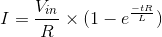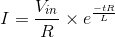# RL Time Constant

## Working principle

In this model, we are showing how the inductor charges with a DC source and then discharges through a resistor. The table below the waveforms displays the time constant of the inductor (the L/R value), the peak current reached during the simulation and the current reached after one time constant (or at 63.2% of the inductor’s total capacity). The user may define the time of the step, which disconnects the source from the inductor and initiates the discharging phase.

### Useful formulas

Charging phase:Discharging phase:### Experiments

• Change the time constant by either increasing or reducing the L/R value and observe the amount of time it takes for the inductor to charge and discharge.
• You may notice that a resistance value of 0 Ohms is not possible here. Why is this?